# Points and Lines

Geometry is a branch of mathematics that deals with drawing various shapes and figures. Before studying geometry, there are some important terms and concepts you need to be familiar with.

Drawing any figure or a shape always starts off from a point. From a point, we start drawing a line and construct various shapes and patterns.

In this mini-lesson we will learn the properties of a point, definitions of a point and a line, how to different types of lines with solved examples and interactive questions.## What Is a Point?

### Definition of a Point

A point is defined as a location in any space. It is represented by a (.)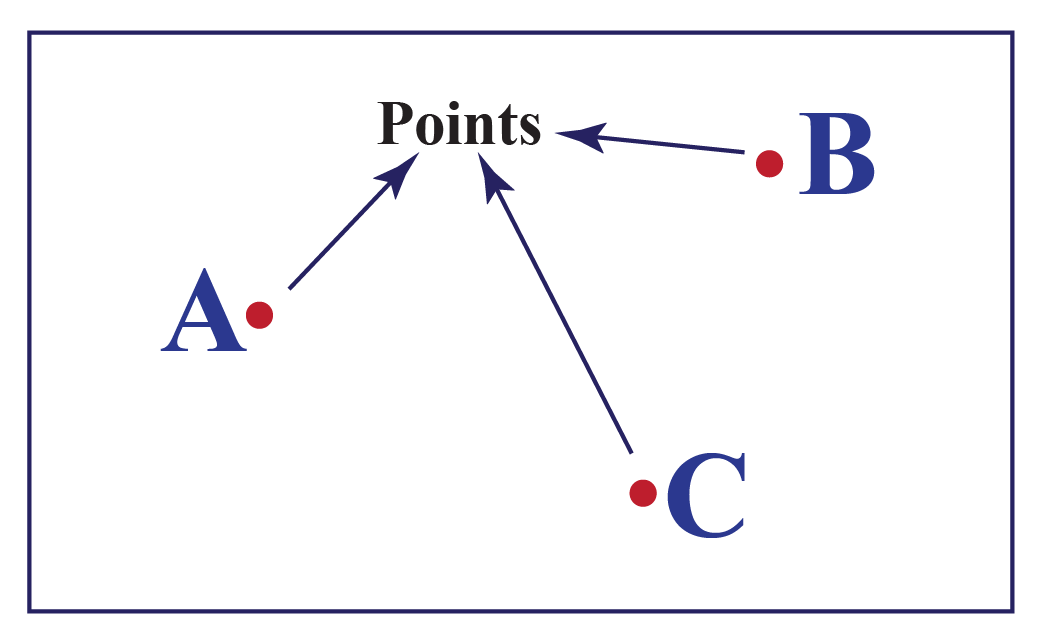### Properties of a Point

• A point does not have any length, height, shape, size.
• It marks the beginning to draw any figure or shape.
• A marked point is labelled by a capital letters

Let us discuss the types of points.

### Collinear Points and Non-Collinear Points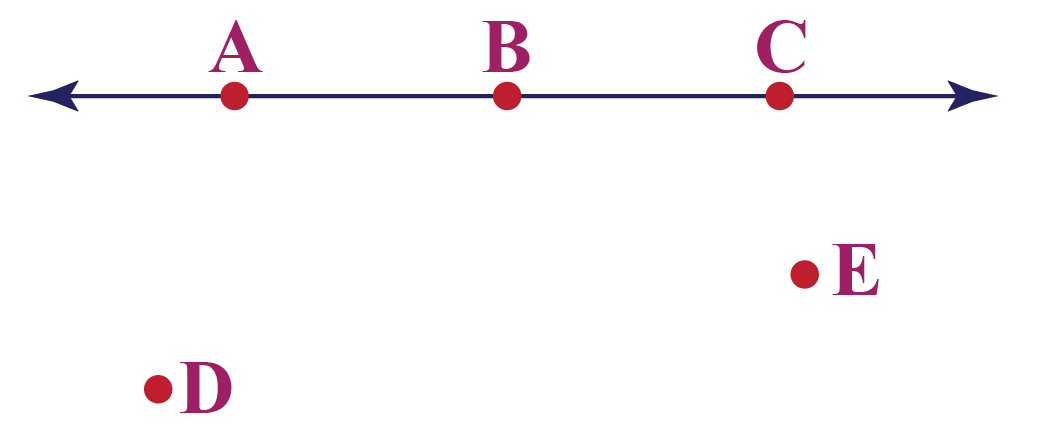If there are three or more points on the same line then they are called collinear points. Here all the points lie on the same line. A,B and C are collinear points.

If there are a group of points that do not lie on the same line then they are called  non-collinear points. D and E are non-collinear points.

### Coplanar Points and Non-Coplanar Points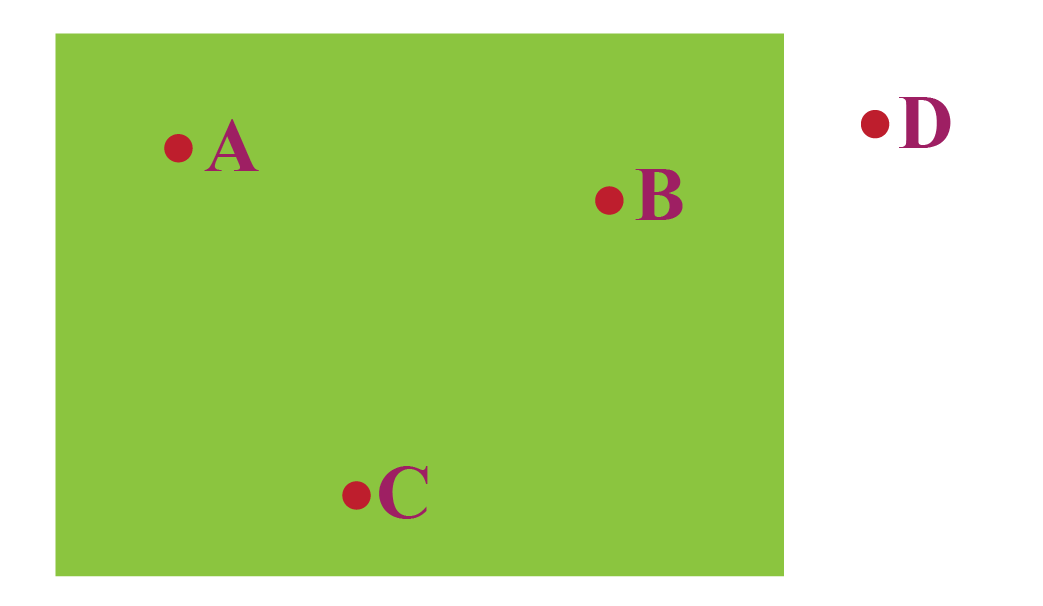If a group of points lie on the same plane then they are said to be coplanar points. A plane is a two-dimensional flat surface that extends infinitely.

A set of points that do not lie on the same plane are non-coplanar points.

### Definition of a Line

A series of points connected by a straight path is defined as a line.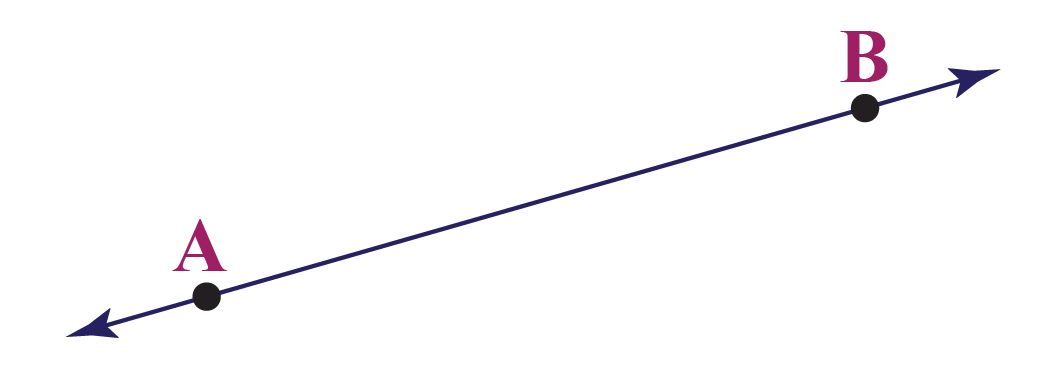### Properties of a Line

• A line is usually defined by two points marked in capital letters
• It extends indefinitely in both the directions
• Length of a line is undefined
• It is denoted by the following notatation \begin{align}\overrightarrow{AB}\end{align}
• There are infinite number of points on a line

Now let us discuss the types of lines.

### Horizontal Line

A line that moves from left to right is called a horizonal line.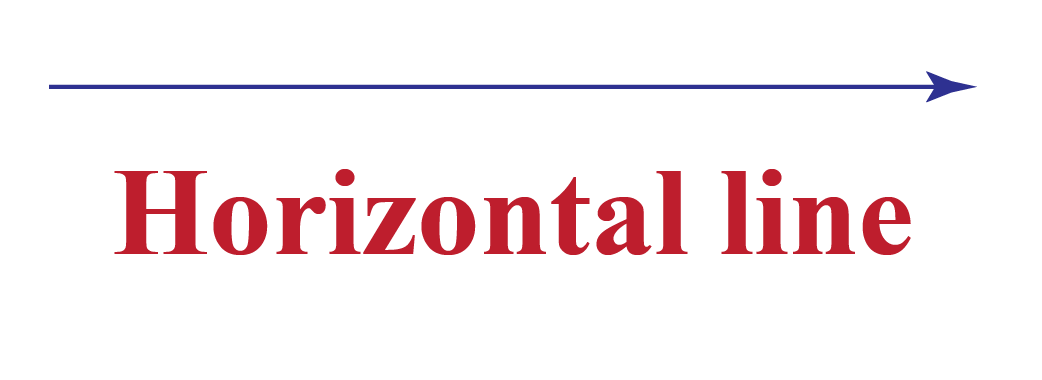### Vertical Line

A line that moves from top to bottom is called a vertical line.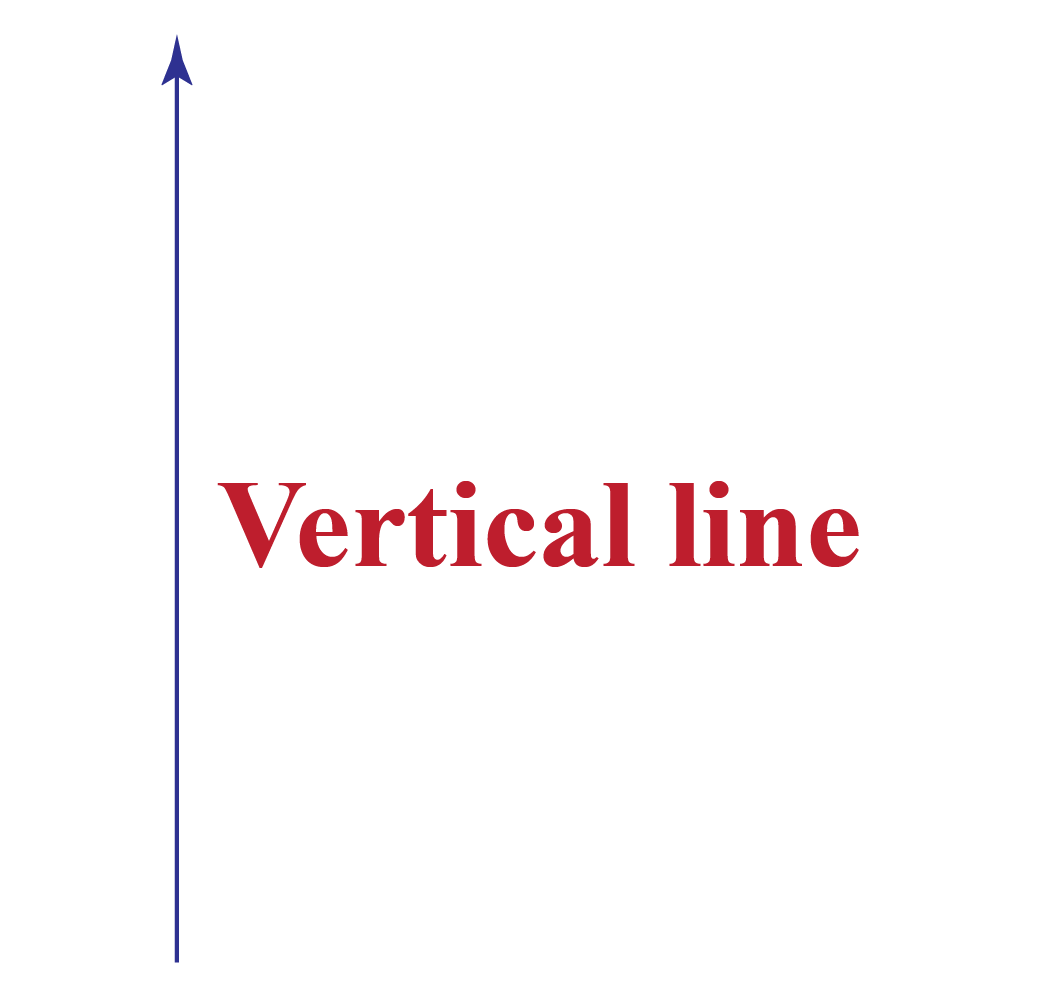### Parallel Line

Two lines are said to be parallel if they do not meet and intersect at any point. The two lines below are said to be parallel lines.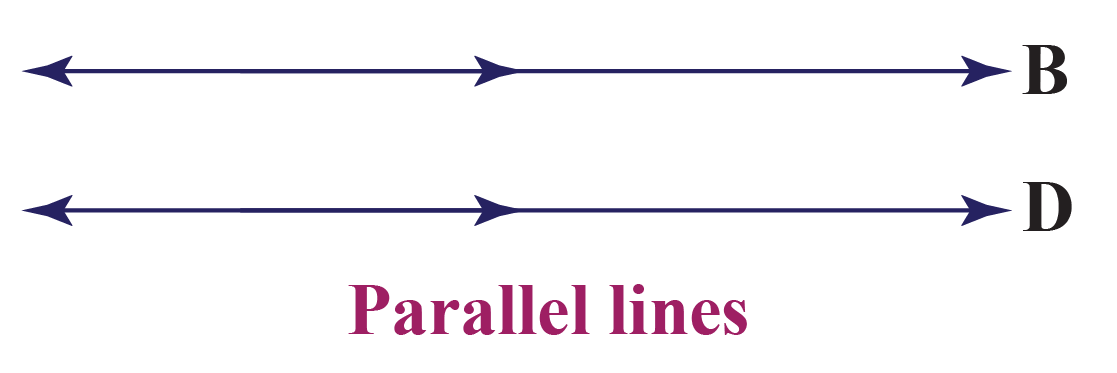### Perpendicular Line

Two lines are said to be perpendicular if they meet or intersect exactly at 900.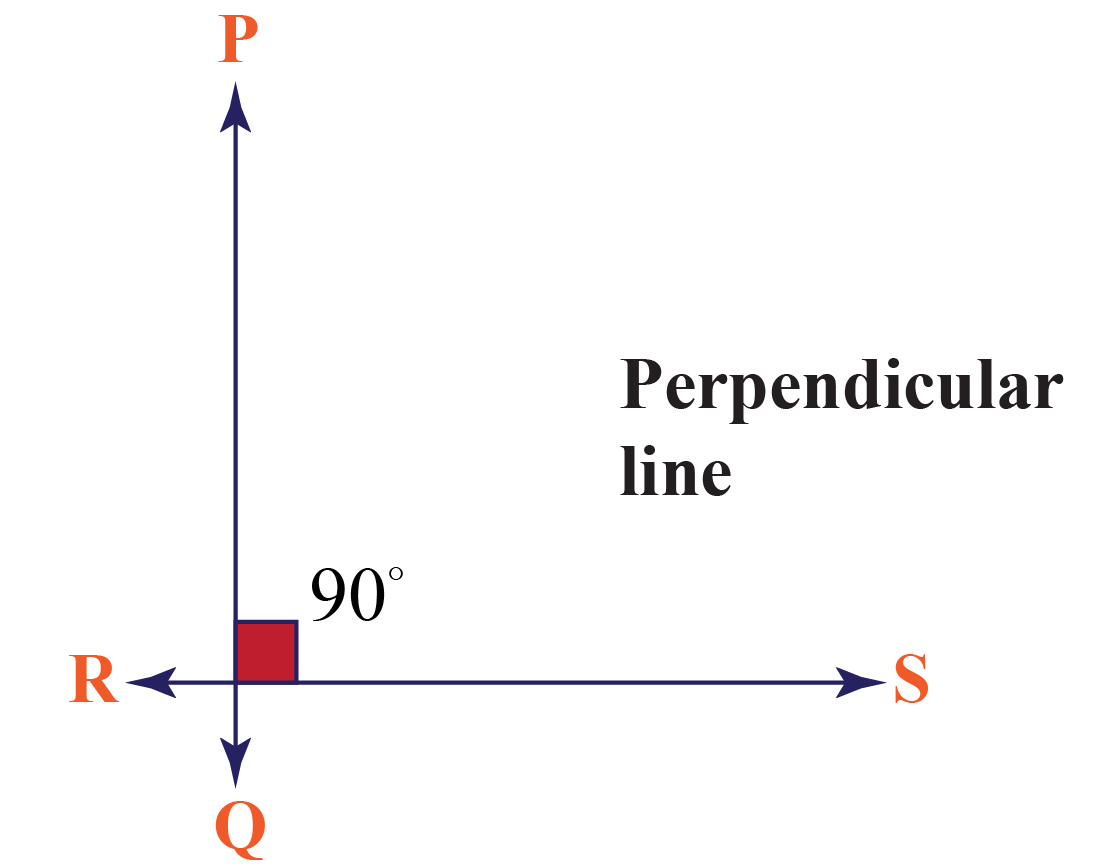### Intersecting Line

Two lines are said to intersect if they meet at a point.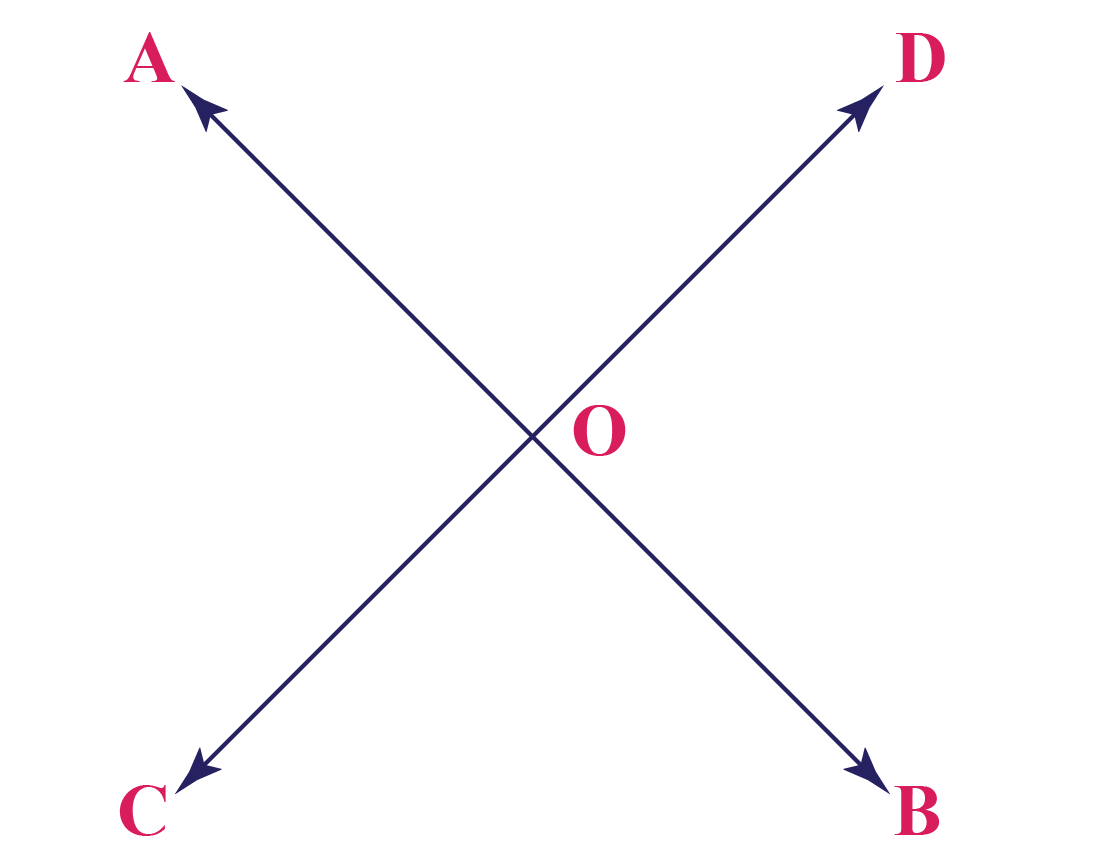## Examples of Points and Lines in Real-World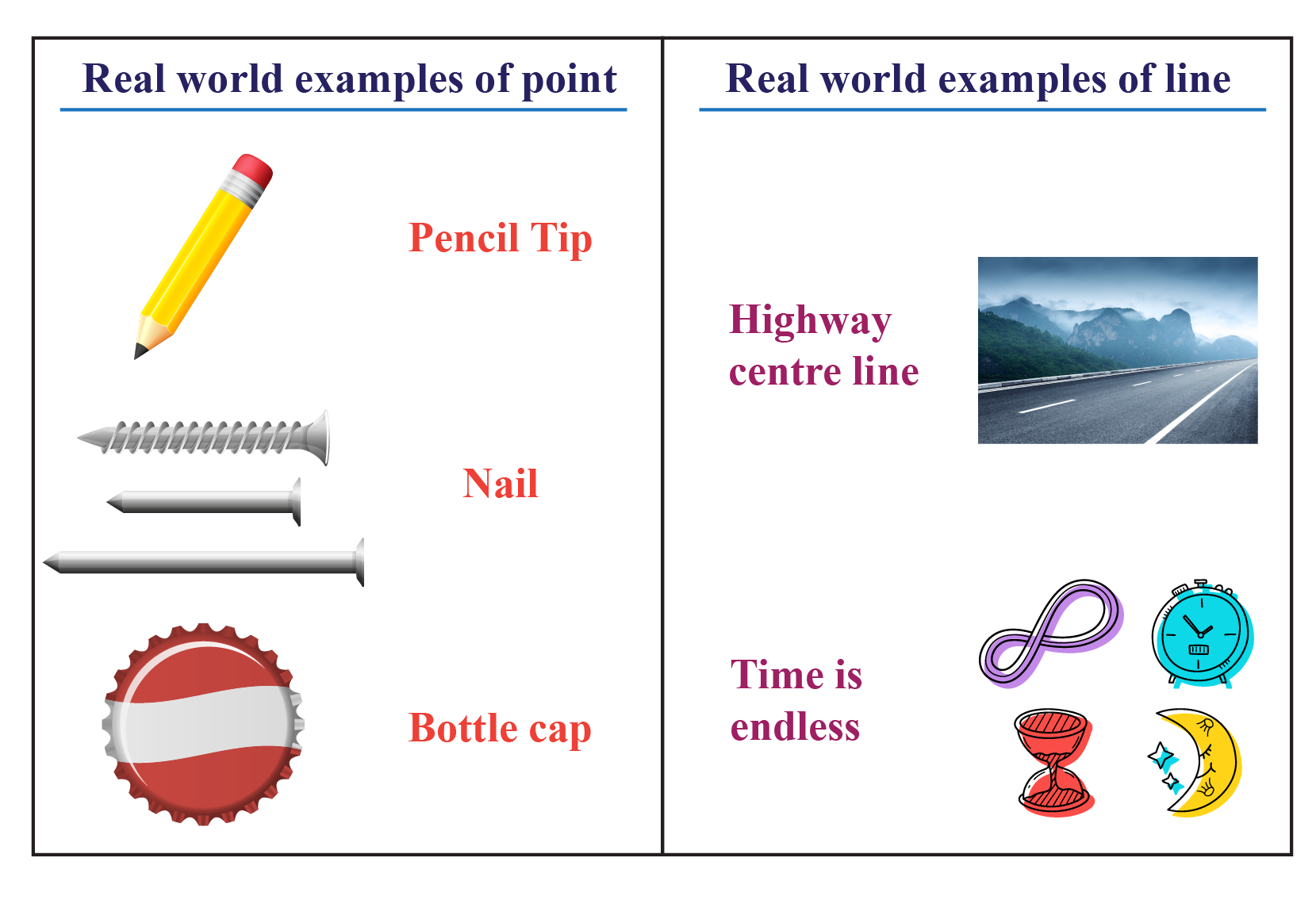Geometry
grade 9 | Questions Set 1
Geometry
grade 9 | Questions Set 2
Geometry